# Eigenvalues of neutral networks: Interpolating between hypercubes

Discrete Mathematics 339, 1283 (2016)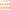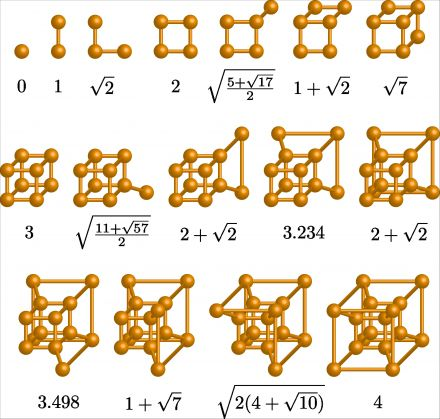The first 16 ‘‘bricklayer’s graphs’’ and the principal eigenvalue of their adjacency matrices.

A neutral network is a subgraph of a Hamming graph, and its principal eigenvalue determines its robustness: the ability of a population evolving on it to withstand errors. Here we consider the most robust small neutral networks: the graphs that interpolate pointwise between hypercube graphs of consecutive dimension (the point, line, line and point in the square, square, square and point in the cube, and so on). We prove that the principal eigenvalue of the adjacency matrix of these graphs is bounded by the logarithm of the number of vertices, and we conjecture an analogous result for Hamming graphs of alphabet size greater than two.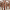#### Imaginary replica analysis of loopy regular random graphs

F. Lopez, T. Coolen

Sub. to Journal of Physics A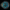#### Taming complexity

M. Reeves, S. Levin, T. Fink, A. Levina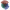#### The mathematical structure of innovation

T. Fink, I. Teimouri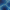#### Replica analysis of Bayesian data clustering

A. Mozeika,, A. Coolen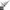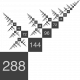#### Recursively divisible numbers

T. Fink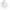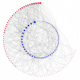#### Degree-correlations in a bursting dynamic network model

F. Vanni, P. Barucca

Journal of Economic Interaction and Coordination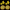#### Phase transition creates the geometry of the continuum from discrete space

R. Farr, T. Fink

Physical Review E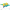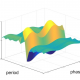#### Intelligently chosen interventions have potential to outperform the diode bridge in power conditioning

F. Liu, Y. Zhang, O. Dahlsten, F. Wang

Scientific Reports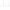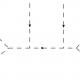#### Scale of non-locality for a system of n particles

S. Talaganis, I. Teimouri

Sub. to Physical Review D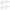#### How much can we influence the rate of innovation?

T. Fink, M. Reeves